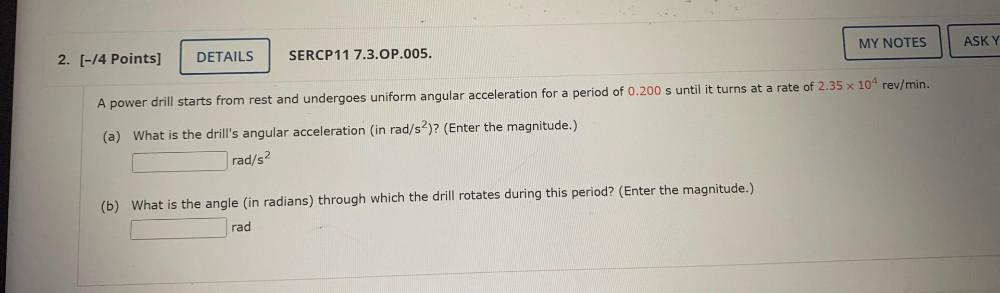Question:

# A power drill starts from rest and undergoes uniform angular acceleration for a period of 0.200 s until it turns at a rate of 2.35 x 10⁴ rev/min. (a) What is the drill's angular acceleration (in rad/sA power drill starts from rest and undergoes uniform angular acceleration for a period of 0.200 s until it turns at a rate of 2.35 x 10⁴ rev/min. (a) What is the drill's angular acceleration (in rad/s²)? (Enter the magnitude.) (b) What is the angle (in radians) through which the drill rotates during this period? (Enter the magnitude.)